Courses

# RD Sharma Solutions - Ex-1.2 Integers, Class 7, Math Class 7 Notes | EduRev

## Class 7: RD Sharma Solutions - Ex-1.2 Integers, Class 7, Math Class 7 Notes | EduRev

The document RD Sharma Solutions - Ex-1.2 Integers, Class 7, Math Class 7 Notes | EduRev is a part of the Class 7 Course RD Sharma Solutions for Class 7 Mathematics.
All you need of Class 7 at this link: Class 7

#### QUESTION 1:

Divide:
(i) 102 by 17
(ii) −85 by 5
(iii) −161 by −23
(iv) 76 by −19
(v) 17654 by −17654
(vi) (−729) by (−27)
(vii) 21590 by −10
(viii) 0 by −135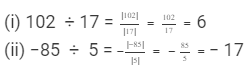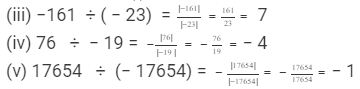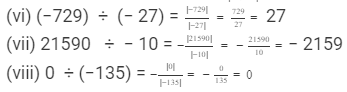#### QUESTION 2:

Fill in the blanks:
(i) 296 ÷ ... = −148
(ii) −88 ÷ ... = 11
(iii) 84 ÷ ... = 12
(iv) ....... ÷ −5 = 25
(v) ....... ÷ 156 = −2
(vi) ....... ÷ 567 = −1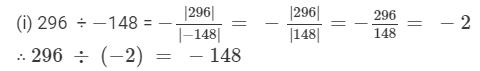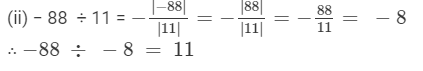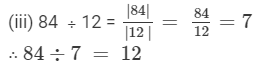(iv) 25×(5) = 12525×(-5) = -125
∴ 125÷5 = 25-125÷-5 = 25
(v) 156×(2) = 312156×(-2) = -312
∴ 312÷156 = 2-312÷156 = -2
(vi) 567×(1) = 567567×(-1) = -567
∴ 567 ÷ 567 = 1

#### QUESTION 3:

Which of the following statements are true?
(i) 0 ÷ 4 = 0
(ii) 0 ÷ (−7) = 0
(iii) −15 ÷ 0 = 0
(iv) 0 ÷ 0 = 0
(v) (−8) ÷ (−1) = −8
(vi) −8 ÷ (−2) = 4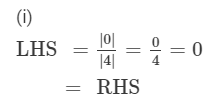Because LHS is equal to RHS, the equation is true.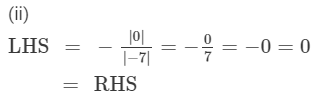Because LHS is equal to RHS, the equation is true.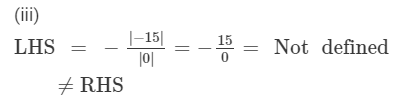Because LHS is not equal to RHS, the equation is false.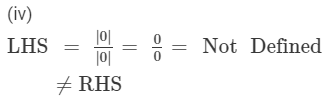Because LHS is not equal to RHS, the equation is false.Because LHS and RHS are not equal, the equation is false.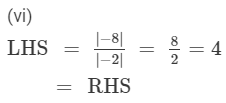Because LHS is equal to RHS, the equation is true.

The document RD Sharma Solutions - Ex-1.2 Integers, Class 7, Math Class 7 Notes | EduRev is a part of the Class 7 Course RD Sharma Solutions for Class 7 Mathematics.
All you need of Class 7 at this link: Class 7Use Code STAYHOME200 and get INR 200 additional OFF Use Coupon Code
All Tests, Videos & Notes of Class 7: Class 7

### Top Courses for Class 7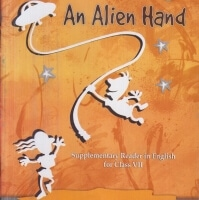## RD Sharma Solutions for Class 7 Mathematics

97 docs

### Top Courses for Class 7Track your progress, build streaks, highlight & save important lessons and more!

,

,

,

,

,

,

,

,

,

,

,

,

,

,

,

,

,

,

,

,

,

,

,

,

,

,

,

;